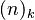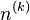# Falling and rising factorials facts for kids

Kids Encyclopedia Facts

The falling factorial$(n)_k$ is a multiplication of progressively smaller terms starting with n where there are k number of terms. Each term is a natural number and decreases by 1.

Similarly the rising factorial$n^{(k)}$is a multiplication of progessively larger terms starting with n where there are k number of terms. Each term is a natural number and increases by 1.Falling and rising factorials Facts for Kids. Kiddle Encyclopedia.